085-1263404

### My Journal

All things Mathematical
2 Apr 2018
##### Question 1

State the conditions of equilibrium of three forces acting on an extended body in one plane.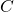is a point vertically above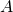, the pivot of light rod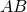, and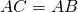.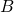is attached totoby a cord and a mass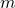is attached to. Show that the thrust on the rod is Independent of the length of the cord and find the tension of the cord whenmakes an angle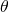with the horizontal.

##### Question 2

State the laws of friction.

A ladder with its centre of gravity at its mid-point rests with one end of the ground and the other against a vertical wall. Show that the greatest inclination to the wall consistent with equilibrium is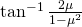where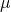is the coefficient of friction both with ground and wall.

##### Question 3

A body revolves with initial angular velocity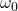and uniform angular acceleration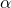: write down equations giving the angular velocity and the angle described in timeand derive and equation not involving.

A wheel is making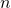revolutions per minute andseconds later it is found to be making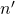revolutions per minute: what is its acceleration (supposed uniform)? How many revolutions has the wheel made in the interval.

##### Question 4

Th resistance to a train weighing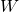tons and travelling at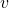miles per hour is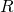lbs. wt. per tone: find the rate of working of the engine.

The train consumes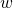tons of coal per hour and the burning oflb. of coal produces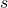ft.-lbs. of energy: what proportion of the energy is usefully employed by the engine?

Evaluate when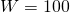,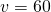,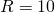,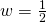,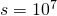.

##### Question 5

The equation of the path of a projectile referred to horizontal and vertical axes is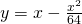; find the angle at which it was projected and the initial velocity. Find also the direction of motion afterseconds. (Note.-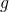may be taken as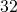).

##### Question 6

A number of unequal particles are distributed in a straight line: find a formula for the position of the centre of gravity. Two masses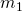and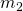are attached to the ends of a light string passing over a smooth peg: show that the acceleration of the centre of gravity is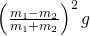.

##### Question 7

A masshangs from a light spiral spring. Show that, ifis pulled down slightly and released, it will move with simple harmonic motion. Find the greatest velocity and the periodic time of, showing clearly on what they depend.

##### Question 8

In a pulley system a weightis raised with uniform acceleration by means of a load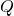: show that the ratio of the accelerations of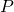andis equal to the velocity ratio of the machine, friction and the weight of the pulleys being neglected.

If in any system of pulleys there is equilibrium when a weightis supported by a load, show that ifbe increased to,will ascend with acceleration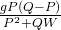.

##### Citation:

State Examinations Commission (2018). State Examination Commission. Accessed at: https://www.examinations.ie/?l=en&mc=au&sc=ru

Malone, D and Murray, H. (2016). Archive of Maths State Exams Papers. Accessed at: http://archive.maths.nuim.ie/staff/dmalone/StateExamPapers/

##### Licence:

“Contains Irish Public Sector Information licensed under a Creative Commons Attribution 4.0 International (CC BY 4.0) licence”.

The EU Directive 2003/98/EC on the re-use of public sector information, its amendment EU Directive 2013/37/EC, its transposed Irish Statutory Instruments S.I. No. 279/2005, S.I No. 103/2008, and S.I. No. 525/2015, and related Circulars issued by the Department of Finance (Circular 32/05), and Department of Public Expenditure and Reform (Circular 16/15 and Circular 12/16).

Note. Circular 12/2016: Licence for Re-Use of Public Sector Information adopts CC-BY as the standard PSI licence, and notes that the open standard licence identified in this Circular supersedes PSI General Licence No: 2005/08/01.